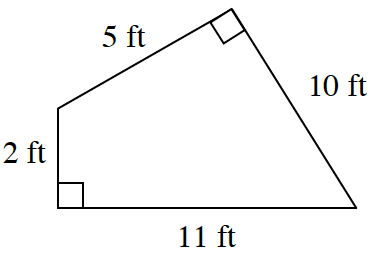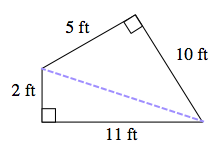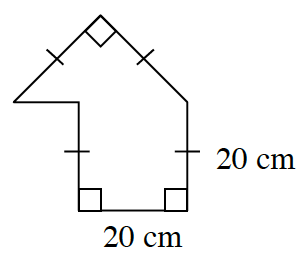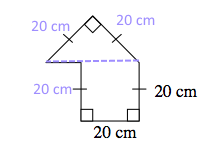### Home > INT2 > Chapter 7 > Lesson 7.2.1 > Problem7-77

7-77.

Determine the area and perimeter of each shape below. Show all work.

1.How can you divide this into easier shapes to find the area?$\text{area}=36 \; \text{ft}^2$
Remember to calculate the perimeter as well.

1.$\text{area}=600 \; \text{cm}^2$
Remember to calculate the perimeter as well.